ChemTalk## Core Concepts

In this article, you will learn how quantity of moles relates to volume, and how to use Avogadro’s Law to determine the volume relation.

## Topics Covered in Other Articles

Avogadro’s law states that the volume of a gas is directly proportional to the number of molecules it contains, provided that the temperature and pressure remain constant. In other words, if you double the number of molecules of a gas, its volume will also double, and if you halve the number of molecules, its volume will be halved. This relationship is described by the equation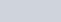, where V1 and V2 are the initial and final volumes of the gas, and n1 and n2 are the initial and final numbers of molecules.

Avogadro’s law is a fundamental principle of chemistry, and it is used to calculate the number of molecules in a given volume of a gas. It takes its name from the Italian scientist Amedeo Avogadro, who first proposed the concept in the early 19th century.

### Important Things to Consider

The gas law described in this article only applies to ideal gases, which you can read about on our article, The Ideal Gas Law.

### Pressure and Moles

Consider a piston filled with a mole of oxygen. The piston has a pressure of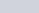. As we know from our article What is Pressure, the pressure of the gas is dependent on the sum of collisions of the gas particles with the walls.

Now, consider what would happen if we added another mole of oxygen to the tank. Now, the amount of particles is doubled, and so the sum of the collisions is also doubled.

From this double in amount of collisions, the force exerted doubles and therefore the pressure also doubles. Since the piston can change in volume to equalize the pressure, the piston expands, according to Boyle’s Law, and the volume increases.

From this, we can start to see the relation between moles and volume.

Avogadro’s Law describes this relationship, which states that the amount of moles and the volume are proportional. This can therefore be described by the equation: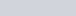.

From Boyle’s Law, we know that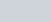. From this relation, we can rewrite Avogadro’s Law as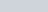.

## Constant Temperature Relation

Because the amount of moles is proportional to the volume, and the amount of moles of a gas in a container isn’t dependent on the identity of the gas, the volume of a certain amount of moles is always the same for a given temperature and pressure.

At standard conditions, for an ideal gas, this volume is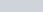. This means that any mole of gas atand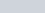will occupy.

Here’s an example of how you can use Avogadro’s Law to solve problems.

A weather balloon becomes filled with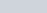of helium gas, and occupies a volume of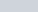. The balloon is overinflated, and therefore the weather engineers designing it have decided to take some helium gas out. The standard size of a weather balloon is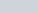. How many moles of helium must you then remove to decrease the volume of the balloon to a safe size?

Solution:Therefore, the engineers must take out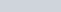of helium gas.

Problem 1

You place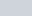of solid CO2 (MW =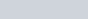), commonly known as dry ice, into an empty balloon. The dry ice then sublimates to gaseous CO2, eventually bringing it to a temperature of. Assuming the balloon had an initial volume of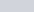, what is its final volume after all the CO2 becomes gas?

Problem 2

Ethanol and methanol combust according to the following chemical equations: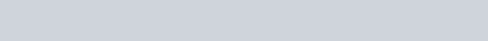You first combust X moles of ethanol and produce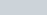of gas. Then, you combust X moles of methanol. What volume of gas do you consequently produce from the methanol combustion?

## Avogadro’s Law Practice Problem Solutions

1:2: